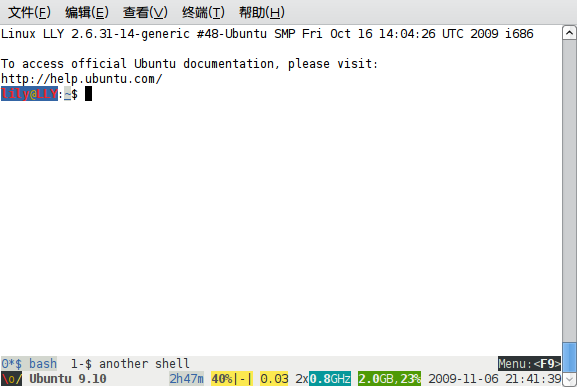# python 内建函数

abs(x)

all(iterable)
iterable里的每项都为真时，才返回真，等效于：

```def all(iterable): for element in iterable: if not element: return False return True```

any(iterable)

```def any(iterable): for element in iterable: if element: return True return False```

basestring()

```>>> isinstance(123, basestring) False >>> isinstance("123", basestring) True >>> isinstance(u"一二三", basestring) True```

bin(x)

bool([x])

callable(object)

chr(i)

classmethod(function)

```class C: @classmethod def f(cls, arg1, arg2, ...): ...```

cmp(x, y)

compile(source, filename, mode[, flags[, dont_inherit]])
source字符串编译成一个AST对象，暂时用不到，先略过。

complex([real[, imag]])

delattr(object, name)

dict([arg])

```>>> dict({"a":"b","c":"d"}) {'a': 'b', 'c': 'd'}```

dir([object])

```>>> dir() ['__builtins__', '__doc__', '__name__', '__package__'] >>> t=[1,2] >>> dir() ['__builtins__', '__doc__', '__name__', '__package__', 't'] >>> dir(t) ['__add__', '__class__', '__contains__', '__delattr__', '__delitem__', '__delslice__', '__doc__', '__eq__', '__format__', '__ge__', '__getattribute__', '__getitem__', '__getslice__', '__gt__', '__hash__', '__iadd__', '__imul__', '__init__', '__iter__', '__le__', '__len__', '__lt__', '__mul__', '__ne__', '__new__', '__reduce__', '__reduce_ex__', '__repr__', '__reversed__', '__rmul__', '__setattr__', '__setitem__', '__setslice__', '__sizeof__', '__str__', '__subclasshook__', 'append', 'count', 'extend', 'index', 'insert', 'pop', 'remove', 'reverse', 'sort']```

divmod(a, b)

enumerate(sequence[, start=0])

```>>> enu=enumerate(['Spring', 'Summer', 'Fall', 'Winter']) >>> enu.next() (0, 'Spring') >>> enu.next() (1, 'Summer') >>> for i, season in enu: ... print i, season ... 2 Fall 3 Winter```

eval(expression[, globals[, locals]])

execfile(filename[, globals[, locals]])

globalslocals的用法和上面的eval同理。

file(filename[, mode[, bufsize]])
File类型的构造函数，参数的作用和下面提到的open()函数是一样的。

filter(function, iterable)

```>>> filter(lambda c: c in 'abc', 'abcdcba') 'abccba' >>> filter(lambda i: i < 3, (1, 2, 3, 2, 1)) (1, 2, 2, 1) >>> filter(lambda i: i < 3, [1, 2, 4, 2, 1]) [1, 2, 2, 1]```

float([x])

format(value[, format_spec])

frozenset([iterable])

getattr(object, name[, default])

globals()

hasattr(object, name)

hash(object)

```>>> hash(1) == hash(1.0) True```

help([object])

hex(x)

id(object)

input([prompt])

int([x[, base]])

isinstance(object, classinfo)

```>>> isinstance(1, (int,float) ) True >>> isinstance(1.0, (int,float) ) True >>> isinstance("1.0", (int,float) ) False```

issubclass(class, classinfo)

iter(o[, sentinel])

```with open("mydata.txt") as fp: for line in iter(fp.readline, "STOP"): process_line(line)```

len(s)

list([iterable])

locals()

long([x[, base]])

map(function, iterable, )
iterable里的每项执行function函数，并把结果以一个list的形式返回。如果有3个以上的参数，则后面的参数也需要是可迭代的，map会把额外的参数传给function，例如，这样可以把两个tuple一一相加得到一个list：

```>>> map(lambda x, add: x + add, (2, 4, 5), (1, 3, 6) ) [3, 7, 11]```

```>>> map(lambda x, add: x + add, (2, 4, 5), (1, 3)) Traceback (most recent call last): File "<stdin>", line 1, in <module> File "<stdin>", line 1, in <lambda> TypeError: unsupported operand type(s) for +: 'int' and 'NoneType'```

max(iterable[, args…][, key])

```>>> max("abcd") 'd' >>> max(1, 2, 3) 3```

```>>> max([1, 2, 3, 4], key=lambda x: x % 3) 2```

min(iterable[, args…][, key])

next(iterator[, default])

```>>> a = iter(range(3)) >>> next(a) 0 >>> next(a) 1 >>> next(a) 2 >>> next(a) Traceback (most recent call last): File "<stdin>", line 1, in <module> StopIteration >>> next(a, "No More Item...") 'No More Item...'```

object()

```>>> o = object() >>> dir(o) ['__class__', '__delattr__', '__doc__', '__format__', '__getattribute__', '__hash__', '__init__', '__new__', '__reduce__', '__reduce_ex__', '__repr__', '__setattr__', '__sizeof__', '__str__', '__subclasshook__']```

oct(x)

open(filename[, mode[, bufsize]])

filename参数，是要打开的文件名。
mode参数是打开方式，通常是`'r'`表示读，`'w'`表示写（如果已存在则会覆盖），`'a'`表示追加。缺省为`'r'`。另外，缺省使用的是文本模式，会把`'\n'`转成系统相关的换行符，如果要避免这个引起的问题，需要在各个模式后面加一个`'b'`表示使用二进制模式。另外还有些’+uU’之类的模式，不常用，也就不介绍了吧。

ord(c)

pow(x, y[, z])

print([object, ][, sep=’ ‘][, end=’\n’][, file=sys.stdout])

property([fget[, fset[, fdel[, doc]]]])

```>>> class C(object): ... def __init__(self): ... self._x = None ... def getx(self): ... print "OK. give you:", self._x ... return self._x ... def setx(self, value): ... print "Now x is:", value ... self._x = value ... def delx(self): ... del self._x ... x = property(getx, setx, delx, "I'm the 'x' property.") ... >>> a = C() >>> a.x = 123 Now x is: 123 >>> print a.x OK. give you: 123 123 >>> help(a.x) OK. give you: 123   >>> help(C.x) #这里可以看到I'm the 'x' property.```

range([start], stop[, step])

raw_input([prompt])

reduce(function, iterable[, initializer])

repr(object)

reversed(seq)

```>>> reversed(range(5)) <listreverseiterator object at 0x80a658c> >>> [i for i in reversed(range(5))] [4, 3, 2, 1, 0]```

round(x[, n])

set([iterable])

```>>> l = [1, 2, 3, 2, 4, 3] >>> set(l) set([1, 2, 3, 4]) >>> list(set(l)) [1, 2, 3, 4] >>> ''.join(set("hello")) 'helo'```

setattr(object, name, value)

slice([start], stop[, step])

```>>> range(5)[slice(1, 4, 2)] [1, 3] >>> range(5)[1:4:2] [1, 3]```

sorted(iterable[, cmp[, key[, reverse]]])

cmp是自定义的比较函数，接受两个参数，返回负数表示第一个参数较小，返回0表示两者一样大，返回正数表示第一个参数较大。
key可以理解为每个参数的求值函数。如果提供了key，则在比较前，先对每个先用key进线求职，对结果再进行排序，但是返回的排序后的结果还是之前的值。
reverse如果是True，则按降序排列，默认是从小到大的升序。

```#正常的排序 >>> sorted([1, 5, 3, 4, 6]) [1, 3, 4, 5, 6] #倒序 >>> sorted([1, 5, 3, 4, 6], reverse=True) [6, 5, 4, 3, 1] #提供了key，结果是除3的余数谁最小，谁就排前 >>> sorted([1, 5, 3, 4, 6], key=lambda x: x%3) [3, 6, 1, 4, 5] #用cmp实现的版本 >>> sorted([1, 5, 3, 4, 6], cmp=lambda x,y: x%3 - y%3) [3, 6, 1, 4, 5]```

staticmethod(function)

```class C: @staticmethod def f(arg1, arg2, ...): ...```

str([object])

sum(iterable[, start])
iterablestart做为初值的基础上进行累加。start的默认值为0。

super(type[, object-or-type])

tuple([iterable])

type(object)

```>>> type(1) <type 'int'> >>> type(type(1)) <type 'type'> >>> type(1) == int #非常不建议这样的使用方法。 True >>> isinstance(1,int) #建议这样使用。 True```

type(name, bases, dict)

```>>> class X(object): ... a = 1 ... >>> X = type('X', (object,), dict(a=1))```

unichr(i)

unicode([object[, encoding[, errors]]])

```>>> unicode('我是bones7456', encoding='utf8') u'\u6211\u662fbones7456' >>> print unicode('我是bones7456', encoding='utf8') #可见解码成功 我是bones7456 >>> unicode('我是bones7456') #不指定编码方式，将默认使用ascii解码，失败了。 Traceback (most recent call last): File "<stdin>", line 1, in <module> UnicodeDecodeError: 'ascii' codec can't decode byte 0xe6 in position 0: ordinal not in range(128) >>> unicode('我是bones7456', errors='ignore') #忽略失败，可以得到英文数字部分 u'bones7456' >>> unicode('我是bones7456', errors='replace') #替换的话，会加上一堆？？？哈哈。 u'\ufffd\ufffd\ufffd\ufffd\ufffd\ufffdbones7456' >>> print unicode('我是bones7456', errors='replace') ������bones7456```

vars([object])

xrange([start], stop[, step])

zip([iterable, ])

zip函数返回一个元组的列表，第i个元组，就包含了每个iterable的第i项。如果参数的各iterable不一样长，会别截取到最短的值，这个值也就是结果列表的长度。

```>>> x = [1, 2, 3] >>> y = [4, 5, 6] >>> zipped = zip(x, y) >>> zipped [(1, 4), (2, 5), (3, 6)] >>> x2, y2 = zip(*zipped) >>> x == list(x2) and y == list(y2) True```

__import__(name[, globals[, locals[, fromlist[, level]]]])

# lafilefixer

`lafilefixer --justfixit`

# 全盘升级至ext4文件系统

```tune2fs -O extents,uninit_bg,dir_index /dev/DEV e2fsck -fpDC0 /dev/DEV fsck /dev/DEV```

/home 分区比较大点，时间长一点，但是完全没有问题哦。

PS：由于上述过程需要umount分区，如果你只有一个linux系统，也可以使用liveCD等进行操作。

========20091227 update========

# byobu 美化你的 screen

byobu的意思，是“屏风”。它提供了一系列漂亮的screen配置。所以，这个包之前叫做screen-profile。